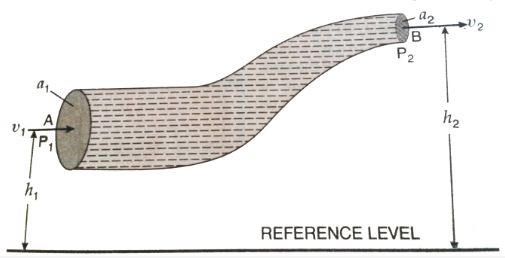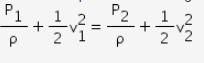# State the limitations and applications of Bernoulli's principle

State the limitations and applications of Bernoulli’s principle

Bernoulli’s equation:Imagine an incompressible and non-viscous liquid to be flowing through a pipe of varying cross-sectional area.When the liquid flows through a horizontal pipe (h1 = h2) then the equation (1) becomesLimitations of Bernoulli’s equation:

1. The above equation has been derived by assuming that the velocity of every element of the liquid across any cross-section of the peipeis uniform. Practically,it is not true. The elements of the liquid in the innermost layer have the maximum velocity. The velocity of the liquid decreases towards the walls of the pipe. Therefore, we should take into account the mean velocity of the liquid.
2. While deriving Bernoulli’s equation, the visous drag of the liquid has not been taken into consideration. The viscous drag comes into play, when a liquid is in motion.
3.Bernoulli’s equation has been derived on the assumption that there appears no loss of energy, when a liquid is in motion. In fsct, some kinetic energy is converted into heat energy and a part of it is lost due to shear force.
3. If the liquid is flowing along a curved path, the energy due to centrifugal force should also be taken into consideration.

Applications of Bernoulli’s theorem :

1. Air foil: Any surface designed so as to obtain reacting force from the air through which it moves is called an air foil.
2. Atomiser or sprayer: An atomiser is used in perfume and deodrant bottles.
3. Blowing of the roofs of the houses during a storm: In a storm, cyclone or hurricane,sometimes the light roofs of the thatchedhouses are blown off.
4. Bunsen’s burner: In Bunsen’s burner, the gas escapesthrough the fine nozzle with a high velocity.
5. Curved path of a spinning ball: When a spinning ball is thrown with a large speed, then the layer of the air moving above the ball gets retarded and the layer below the ball gets accelerated due to the spin motion.
6. Vacuum brake: Vacuum brake is used to stop the railway train in case of emergency.